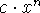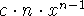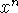CS 2005 Techniques of Programming WPI, B Term 1996
Craig E. Wills Project 1 (20 pts)
Assigned: Thursday, October 31, 1996 Due: Friday, November 8, 1996

# Introduction

This assignment is designed to review your introductory programming skills and be a lead in to our first group project in the course. It builds on the example discussed in class concerning the representation of polynomials.

# Background

Your program will be computing and evaluating the derivative (differential) of a polynomial. You may recall (or will soon find out) that for the polynomial termthe derivative is. The derivative of a constant term (n=0) is zero. The derivative of a entire polynomial function f(x) (commonly expressed as f'(x)) is the sum of the derivatives of the individual terms. Evaluation of the derivative at a particular point gives the slope of f(x) at that point.

Similarly, your program will be computing and evaluating the integral of a polynomial. You may recall (or will soon find out) that for the polynomial termthe integral is. The integral of a entire polynomial function f(x) (commonly expressed as F(x)) is the sum of the integrals of the individual terms. Integration of a polynomial also results in a constant of integration term. For this assignment we will assume that the constant of integration is always zero.

It is common to evaluate an integral over an interval, which simply means to evaluate the integral at two points. The value of the integral at the first point is subtracted from the value of the integral at the second point to obtain the result. This value is the area under the curve of f(x) between the two points.

# Assignment

Your assignment is to build on the polynomial example discussed in class so that you can represent, print, differentiate, integrate and evaluate a polynomial. Your program must be menu driven with the following menu items (you need to use this same numbering mechanism):

```0. Print this menu.
1. Exit.
2. Input a new polynomial.
3. Print the current polynomial.
4. Evaluate the current polynomial at a point and print the result.
5. Differentiate the current polynomial and print the resulting polynomial.
6. Evaluate the differential at a point and print the result.
7. Integrate the current polynomial and print the resulting polynomial.
8. Evaluate the integral over an interval and print the result.```

The program should continually loop, requesting a command (input as an integer) and executing it. You should do error checking. A sample script for your program might be:

```> poly
Enter a command (2-8, 0-help, 1-exit): 2
What is the degree of your polynomial?
3
Enter the coefficient for the degree 3 term: 2
Enter the coefficient for the degree 2 term: 1
Enter the coefficient for the degree 1 term: 3
Enter the coefficient for the degree 0 term: -4

Enter a command (2-8, 0-help, 1-exit): 3
f(x) = + 2x^3 + 1x^2 + 3x^1 + -4x^0

Enter a command (2-8, 0-help, 1-exit): 4
Enter the point for evaluation: 2
f(2) = 22

Enter a command (2-8, 0-help, 1-exit): 5
f'(x) = + 6x^2 + 2x^1 + 3x^0

Enter a command (2-8, 0-help, 1-exit): 6
Enter the point for evaluation: 2
f'(2) = 31

Enter a command (2-8, 0-help, 1-exit): 7
F(x) = + 0.5x^4 + 0.333333x^3 + 1.5x^2 + -4x^1 + 0x^0

Enter a command (2-8, 0-help, 1-exit): 8
Enter the first point of the interval: 0
Enter the second point of the interval: 1
F(1) - F(0) = -1.666667

Enter a command (2-8, 0-help, 1-exit): 1
>```

# Approach

You are encouraged to start with the code discussed in class and available online in
/cs/cs2005/pub/example/poly.C. You should modify the print routine to give ``prettier'' output than used in the class example or the sample script above. You will also need to add an additional parameter to the print routine so it knows whether it is printing f(x), f'(x) or F(x).

In addition to the ReadPoly() and PrintPoly() functions, you should create the following functions with the indicated parameters to differentiate, integrate and evaluate a polynomial. These functions should be used as a basis for carrying out the commands performed by the program. Note: Only the PrintPoly() function should print a polynomial, functions to differentiate or integrate polynomials should not print the computed polynomial.

```/* compute the differential and store it in rgCoeffDiff with the degree in
wDegreeDiff */
void DiffPoly(CoeffList rgCoeff, int wDegree, CoeffList rgCoeffDiff,
int &wDegreeDiff);

/* compute the integral and store it in rgCoeffInt with the degree in
wDegreeInt */
void IntPoly(CoeffList rgCoeff, int wDegree, CoeffList rgCoeffInt,
int &wDegreeInt);

/* evaluate the polynomial at the given point.  Return the value as the
result of the function. */
float EvalPoly(CoeffList rgCoeff, int wDegree, float fPt);```

Note to computeyou can use the function pow(x,n) contained in the standard Unix math library (you need to include the header file `<math.h>` at the beginning of program for the function prototype). It takes two arguments of type double and returns the result as a double. You will need to cast integer types to double types to use it as illustrated in the following.

```    float x,y;
int n;

y = pow(x, double(n));    /* cast integer value to double */```

You will also need to include the math library as part of your compilation using the -lm flag as the last argument of the compilation. Thus you will need to use:

`> g++ -o poly poly.C -lm`

# Submission

The program is due on Friday, November 8. However you will be discussing your program in your group in lab on Wednesday, November 6 and should have as much of your program done as possible at that time. You should bring a copy of your program to lab on that day. Instructions on turning in your program along with needed changes based on your group discussion will be given in lab.